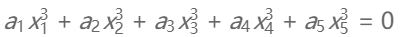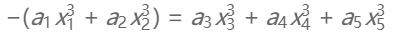# POJ 1840 - Eqs

• POJ 1840 - Eqs
• Time: 5000MS
• Memory: 65536K
• 难度: 初级
• 分类: 高效查找法

## 解题思路## AC 源码

``````//Memory Time
//49188K 532MS

#include<iostream>
using namespace std;

short hash;  //hash[sum]表示值等于sum的的解的个数(多对1映射)

int main(void)
{
int a1,a2,a3,a4,a5;  //系数
while(cin>>a1>>a2>>a3>>a4>>a5)
{
memset(hash,0,sizeof(hash));

for(int x1=-50;x1<=50;x1++)
{
if(!x1)
continue;

for(int x2=-50;x2<=50;x2++)
{
if(!x2)
continue;
int sum=(a1*x1*x1*x1 + a2*x2*x2*x2)*(-1);
if(sum<0)
sum+=25000000;
hash[sum]++;
}
}

int solution=0;

for(int x3=-50;x3<=50;x3++)
{
if(!x3)
continue;
for(int x4=-50;x4<=50;x4++)
{
if(!x4)
continue;
for(int x5=-50;x5<=50;x5++)
{
if(!x5)
continue;
int sum=a3*x3*x3*x3 + a4*x4*x4*x4 + a5*x5*x5*x5;
if(sum<0)
sum+=25000000;
if(hash[sum])
solution+=hash[sum];
}
}
}

cout<<solution<<endl;
}
return 0;
}``````

目录